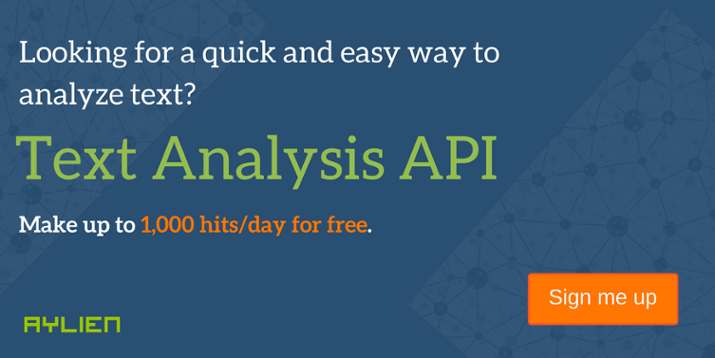# Naive Bayes for Dummies; A Simple Explanation

Commonly used in Machine Learning, Naive Bayes is a collection of classification algorithms based on Bayes Theorem. It is not a single algorithm but a family of algorithms that all share a common principle, that every feature being classified is independent of the value of any other feature. So for example, a fruit may be considered to be an apple if it is red, round, and about 3″ in diameter. A Naive Bayes classifier considers each of these “features” (red, round, 3” in diameter) to contribute independently to the probability that the fruit is an apple, regardless of any correlations between features. Features, however, aren’t always independent which is often seen as a shortcoming of the Naive Bayes algorithm and this is why it’s labeled “naive”.

Although it’s a relatively simple idea, Naive Bayes can often outperform other more sophisticated algorithms and is extremely useful in common applications like spam detection and document classification.

In a nutshell, the algorithm allows us to predict a class, given a set of features using probability. So in another fruit example, we could predict whether a fruit is an apple, orange or banana (class) based on its colour, shape etc (features).

### Pros and cons of Naive Bayes:

• It’s relatively simple to understand and build
• It’s easily trained, even with a small dataset
• It’s fast!
• It’s not sensitive to irrelevant features

• It assumes every feature is independent, which isn’t always the case

### Explanation:

A simple example best explains the application of Naive Bayes for classification. When writing this blog I came across many examples of Naive Bayes in action. Some were too complicated, some dealt with more than Naive Bayes and used other related algorithms, but we found a really simple example on StackOverflow which we’ll run through in this blog. It explains the concept really well and runs through the simple maths behind it without getting too technical.

So, let’s say we have data on 1000 pieces of fruit. The fruit being a Banana, Orange or some Other fruit and imagine we know 3 features of each fruit, whether it’s long or not, sweet or not and yellow or not, as displayed in the table below:

So from the table what do we already know?

• 50% of the fruits are bananas
• 30% are oranges
• 20% are other fruits

Based on our training set we can also say the following:

• From 500 bananas 400 (0.8) are Long, 350 (0.7) are Sweet and 450 (0.9) are Yellow
• Out of 300 oranges 0 are Long, 150 (0.5) are Sweet and 300 (1) are Yellow
• From the remaining 200 fruits, 100 (0.5) are Long, 150 (0.75) are Sweet and 50 (0.25) are Yellow

Which should provide enough evidence to predict the class of another fruit as it’s introduced.

So let’s say we’re given the features of a piece of fruit and we need to predict the class. If we’re told that the additional fruit is Long, Sweet and Yellow, we can classify it using the following formula and subbing in the values for each outcome, whether it’s a Banana, an Orange or Other Fruit. The one with the highest probability (score) being the winner.

#### Banana:

$$\ P(Banana|Long,Sweet,Yellow) = frac{P(Long|Banana) cdot P(Sweet|Banana) cdot P(Yellow|Banana) cdot P(Banana)}{ P(Long) cdot P(Sweet) cdot P(Yellow)}$$

$$\ = frac{0.8 times 0.7 times 0.9 times 0.5}{P(evidence)}$$

$$\ = frac{0.252}{P(evidence)}$$

#### Orange:

$$\ P(Orange|Long,Sweet,Yellow) = 0$$

#### Other Fruit:

$$\ P(Other|Long,Sweet,Yellow) = frac{P(Long|Other) cdot P(Sweet|Other) cdot P(Yellow|Other) cdot P(Other)}{ P(Long) cdot P(Sweet) cdot P(Yellow)}$$

$$\ = frac{0.5 times 0.75 times 0.25 times 0.2}{P(evidence)}$$

$$\ = frac{0.01875}{P(evidence)}$$

In this case, based on the higher score (0.01875 lt 0.252) we can assume this Long, Sweet and Yellow fruit is, in fact, a Banana.

Now that we’ve seen a basic example of Naive Bayes in action, you can easily see how it can be applied to Text Classification problems such as spam detection, sentiment analysis and categorization. By looking at documents as a set of words, which would represent features, and labels (e.g. “spam” and “ham” in case of spam detection) as classes we can start to classify documents and text automatically. You can read more about Text Classification in our Text Analysis 101 Series.

There you have it, a simple explanation of Naive Bayes along with an example. We hope this helps you get your head around this simple but common classifying method.Let's Talk